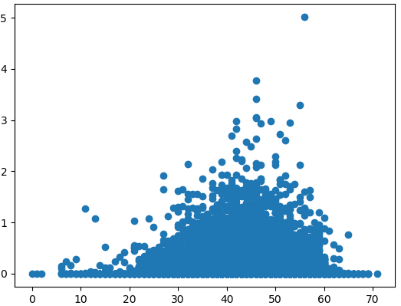# Matplotlib: Create a Plot Using plt.scatter()

In this tutorial, we will introduce how to create a plot using plt.scatter() in matplotlib.```import matplotlib.pyplot as plt
import pandas as pd

In this code, we will use pandas to read a csv file.

Run this code, you will see:

```         DATE  PRCP  TMAX  TMIN  RAIN
0  1948-01-01  0.47    51    42  True
1  1948-01-02  0.59    45    36  True
2  1948-01-03  0.42    45    35  True
3  1948-01-04  0.31    45    34  True
4  1948-01-05  0.17    45    32  True```

3.Create a plot using plt.scatter()

```PRCP = weather_data['PRCP']
TMAX = weather_data['TMAX']
TMIN = weather_data['TMIN']
plt.scatter(TMIN, PRCP)
plt.show()```

Run this code, you will see this plot.